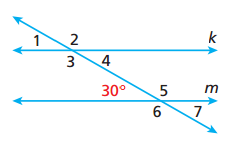# Types of Angles

Topic:
Angles

## Complementary Angles

1) What do you notice about the two angles when you slide the two sliders? What do complementary angles add up to?

## Supplementary Angles

2) What do you notice about these two angles? What do supplementary angles add up to?

## Hmmm.....

3) What is the complementary angle to 38? 4) What is the supplementary angle to 38? 5) Is it possible for one angle of a pair of complementary angles to be 100? Why or why not?

## Vertical Angles

Vertical angles are angles that are across from each other when two lines intersect. 6) What do you notice about the angle measures of vertical angles?

## Transversal

A transversal is a line that intersects two parallel lines. 7) First check the "Show Corresponding Angles" box. What do you notice as you slide the first slider? Corresponding angles are in the SAME SPOT on different parallel lines. On your paper write, "Corresponding angles are in the same spot on different parallel lines." 8) Now, check the "Show Alternate Interior Angles" box. What do you notice as you slide the first slider? Alternate Interior Angles show the opposite angles on the inside of parallel lines. They have the same angle measure. On your paper, write how you will remember Alternate Interior Angles. 9) Lastly, check the "Show Alternate Exterior Angles" box. What do you notice as you slide the first slider? Alternate Exterior Angles show the opposite angles on the outside of the parallel lines. They have the same angle measure. On your paper, write how you will remember the difference between Alternate Interior Angles and Alternate Exterior Angles.

## Example!Now that you have notes on the different types of angles made by a transversal, find the missing angle measures.
10) m1 = m2= m3= m4= m5= m6= m7=